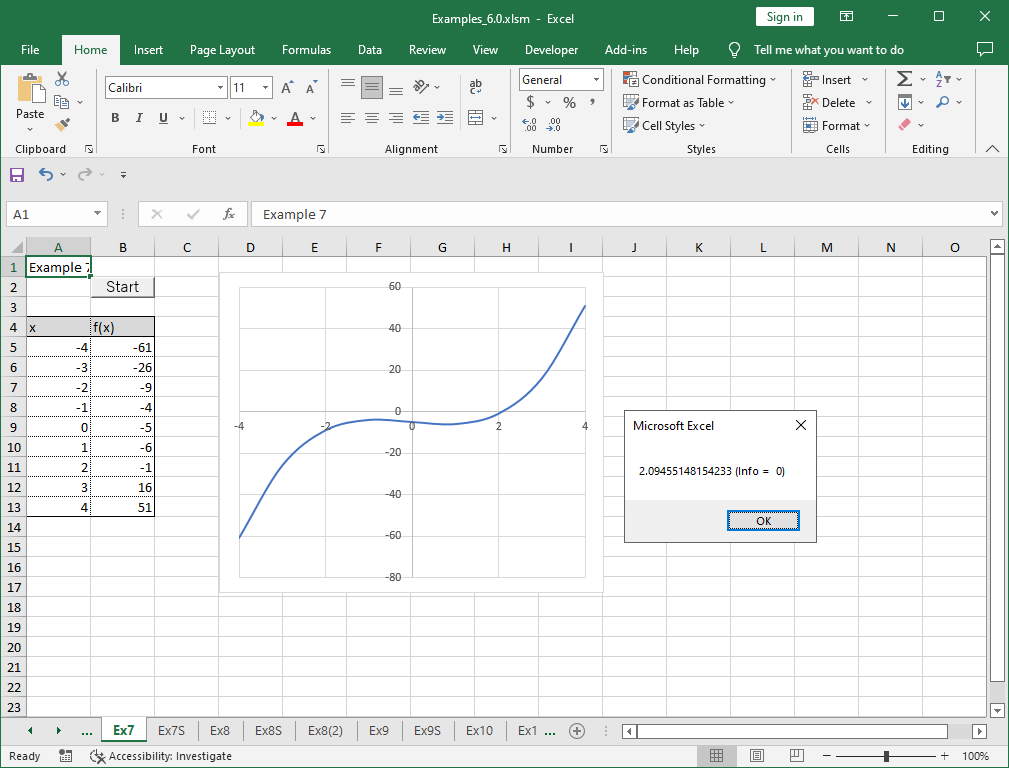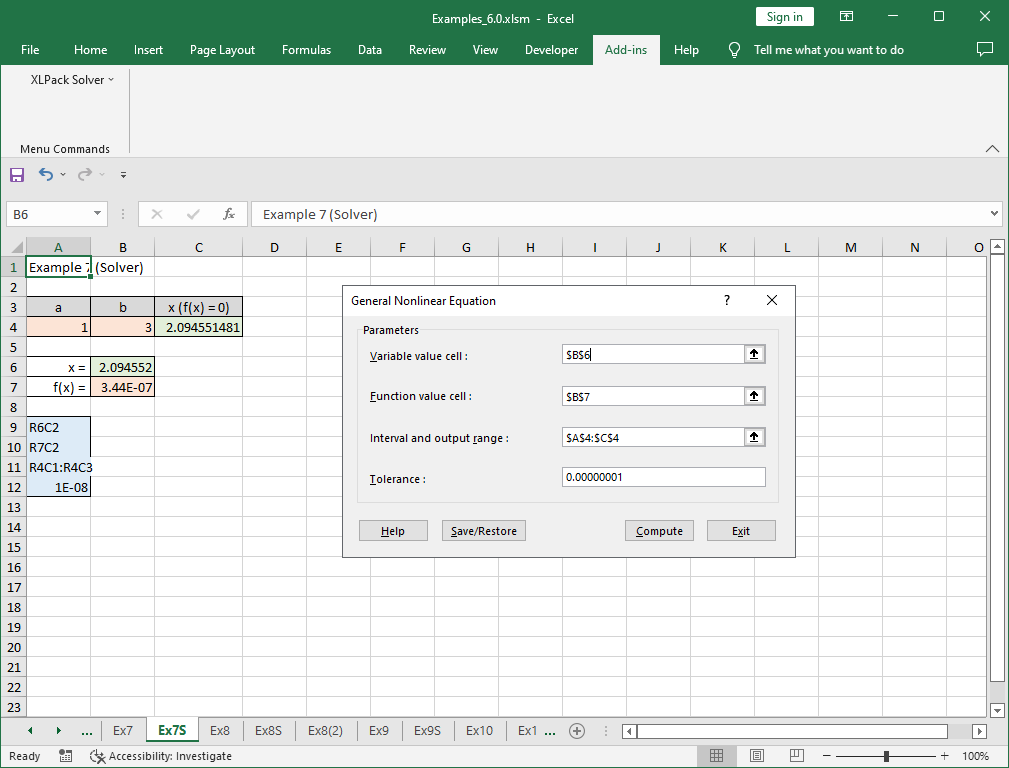# 7. Solution of nonlinear equation

Note – The example worksheets are available for download. See 1. How to obtain and use example worksheets.

Examples of solving a single variable nonlinear equation, i.e. finding a root of arbitrary one-dimensional nonlinear function by XLPack are shown below.

### Example

Now, we consider the following equation.

```
f(x) = x3 - 2x - 5 = 0

```

To check the shape of f(x), let’s draw the graph of the function.Since we see that zero point is located near x = 2 from the graph, we will use [1, 3] as the interval including zero.

### Solving problem with VBA program (1)

The following is an example code using VBA library subroutine Dfzero.

``````Function F(X As Double) As Double
F = X ^ 3 - 2 * X - 5
End Function

Sub Start()
Dim Ax As Double, Bx As Double, Rx As Double, Re As Double, Ae As Double, Info As Long
'--- Input data
Ax = 1
Bx = 3
Rx = Ax
Re = 0.000000000000001 '1e-15
Ae = Re
'--- Compute zero of equation
Call Dfzero(AddressOf F, Ax, Bx, Rx, Re, Ae, Info)
'--- Output zero
MsgBox Str(Ax) & " (Info = " & Str(Info) & ")"
End Sub``````

You need to define the object function as the external VBA function, and call Dfzero with specifying the interval and the tolerance. Please make sure that the supplied interval includes a zero.

The result X = 2.0945514815 is obtained by running this program.### Solving problem with VBA program (2)

The following is an example code using the reverse communication version VBA library subroutine Dfzero_r.

``````Sub Start()
Dim Ax As Double, Bx As Double, Rx As Double, Re As Double, Ae As Double, Info As Long
Dim XX As Double, YY As Double, IRev As Long
'--- Input data
Ax = 1
Bx = 3
Rx = Ax
Re = 0.000000000000001 '1e-15
Ae = Re
'--- Compute zero of equation
IRev = 0
Do
Call Dfzero_r(Ax, Bx, Rx, Re, Ae, Info, XX, YY, IRev)
If IRev <> 0 Then YY = XX ^ 3 - 2 * XX - 5
Loop While IRev <> 0
'--- Output zero
MsgBox Str(Ax) & " (Info = " & Str(Info) & ")"
End Sub``````

Instead of defining the object function as the external VBA function, when returned with IRev = 1, you need to compute the object function value at XX, enter it in YY and call Dfzero_r again. For further details of reverse communication version, see here.

The same result with above is obtained by this program.

### Solving problem with Solver

“General Nonlinear Equation” of XLPack solver add-in can be used to solve this problem. The formula (=B6^3-2*B6-5) is entered in cell B7.Please refer to here for how to use solver.